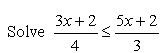Email us to get an instant 20% discount on highly effective K-12 Math & English kwizNET Programs!

#### Online Quiz (WorksheetABCD)

Questions Per Quiz = 2 4 6 8 10

### Middle/High School Algebra, Geometry, and Statistics (AGS)2.17 Inequation Review Test

 Q 1: Solve 5(x + 4) < 9(x - 4).x < 13x > 14x < 14x > 15 Q 2:x < 1/3x > 19/11x < 2/3x > 11/19 Q 3: The point (4,5) ____ the line 2x - y = 3.does not satisfysatisfies Q 4:x greater than or equal to - 2/11x less than or equal to 2/11 Q 5: The origin (0,0)_____ the line y < 2x + 1.does not belongsbelongs Q 6:x greater than or equal to 11x greater than or equal to -11 Q 7: The line y = x + 2 is the boundary line for the region y Ł x + 2. FalseTrue Q 8: The line y = 2x + 1 is the boundary line for the region y < 2x + 1.TrueFalse Question 9: This question is available to subscribers only! Question 10: This question is available to subscribers only!

#### Subscription to kwizNET Learning System offers the following benefits:

• Unrestricted access to grade appropriate lessons, quizzes, & printable worksheets
• Instant scoring of online quizzes
• Progress tracking and award certificates to keep your student motivated
• Unlimited practice with auto-generated 'WIZ MATH' quizzes